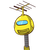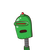# 9. The cost of 3= kg of oranges is 91. Find the cost of 1 kg oranges.​

9. The cost of 3= kg of oranges is 91. Find the cost of 1 kg oranges.​

### 2 thoughts on “9. The cost of 3= kg of oranges is 91. Find the cost of 1 kg oranges.​”

1.THE COST OF 1 KG ORANGE IS 30.33

Step-by-step explanation:

COST OF 3 KG ORANGES IS = 91

COST OF 1 KG ORANGE IS = 91/3

= 30.33

2.